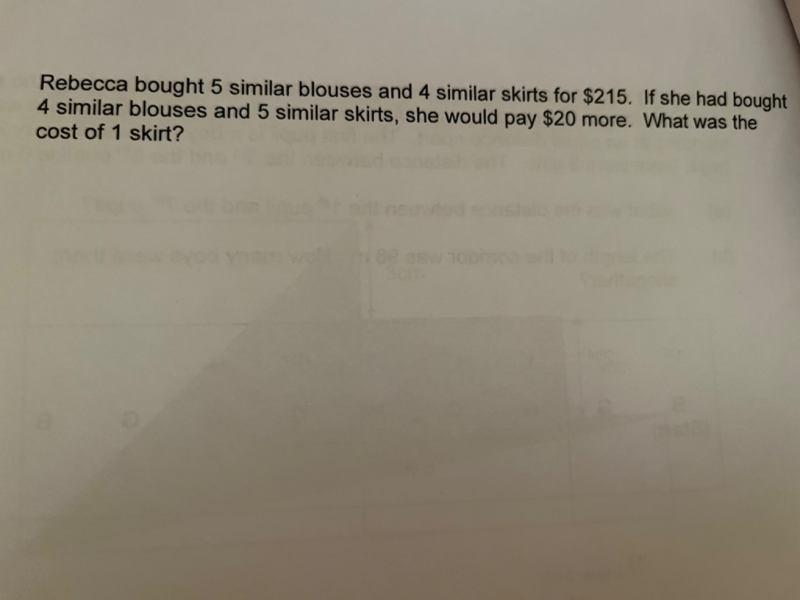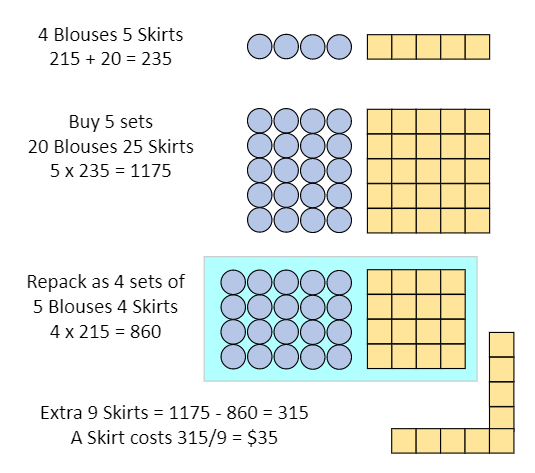# QuestionSource: Woodgrove Primary

This old question resurfaced to the top for some weird reason.

Anyway, here is a non-algebraic solution using block diagram. (Underlying principle is algebra but I think maybe P5s understand block diagrams better than equations with 2 unknowns.)0 Replies 1 Like

Oops… so I did… * embarrassed *

Thanks for finding the mistake!

Let’s do it again:

Let B be the cost of a blouse.
Let S be the cost of a skirt.

5 B + 4 S = 215 … (1)

4 B + 5 S = 215 + 20 = 235
=> 5 B + (5/4)5 S = (5/4)235
=> 5 B + (25/4) S = 293.75 … (2)

(2) – (1) :
(9/4) S = 293.75 – 215 = 78.75
S = 78.75 (4/9) = \$35 #

0 Replies 0 Likes